Courses

# The Multistory Frames with Sidesway - 1 Civil Engineering (CE) Notes | EduRev

## Civil Engineering (CE) : The Multistory Frames with Sidesway - 1 Civil Engineering (CE) Notes | EduRev

The document The Multistory Frames with Sidesway - 1 Civil Engineering (CE) Notes | EduRev is a part of the Civil Engineering (CE) Course Structural Analysis.
All you need of Civil Engineering (CE) at this link: Civil Engineering (CE)

Instructional Objectives

After reading this chapter the student will be able to
1. Identify the number of independent rotational degrees of freedom of a rigid frame.
2. Write appropriate number of equilibrium equations to solve rigid frame having more than one rotational degree of freedom.
3. Draw free-body diagram of multistory frames.
4. Analyse multistory frames with sidesway by the slope-deflection method.
5. Analyse multistory frames with sidesway by the moment-distribution method.

Introduction

In lessons 17 and 21, rigid frames having single independent member rotational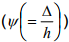degree of freedom (or joint translation Δ) is solved using slopedeflection and moment-distribution method respectively. However multistory frames usually have more than one independent rotational degree of freedom. Such frames can also be analysed by slope-deflection and moment-distribution methods. Usually number of independent member rotations can be evaluated by inspection. However if the structure is complex the following method may be adopted. Consider the structure shown in Fig. 22.1a. Temporarily replace all rigid joints of the frame by pinned joint and fixed supports by hinged supports as shown in Fig. 22.1b. Now inspect the stability of the modified structure. If one or more joints are free to translate without any resistance then the structure is geometrically unstable. Now introduce forces in appropriate directions to the structure so as to make it stable. The number of such externally applied forces represents the number of independent member rotations in the structure.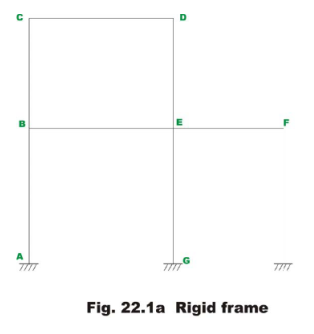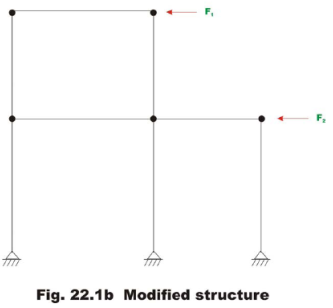In the modified structure Fig. 22.1b, two forces are required to be applied at level CD and level BF for stability of the structure. Hence there are two independent member rotations (ψ) that need to be considered apart from joint rotations in the analysis.

The number of independent rotations to be considered for the frame shown in Fig. 22.2a is three and is clear from the modified structure shown in Fig. 22.2b.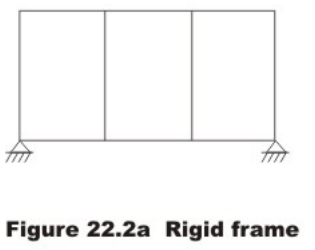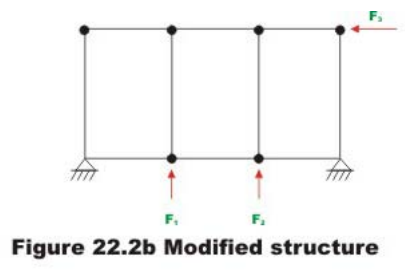From the above procedure it is clear that the frame shown in Fig. 22.3a has three independent member rotations and frame shown in Fig 22.4a has two independent member rotations.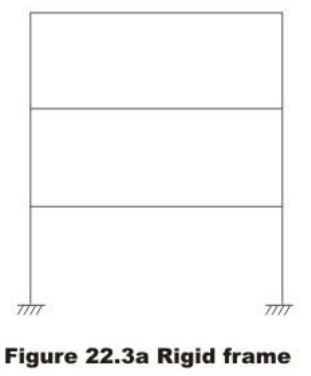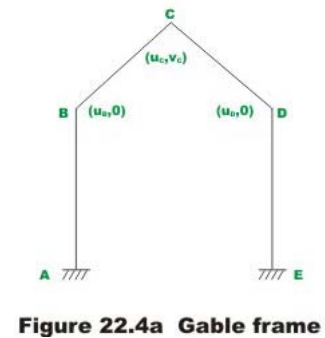For the gable frame shown in Fig. 22.4a, the possible displacements at each joint are also shown. Horizontal displacement is denoted by u and vertical displacement is denoted by v . Recall that in the analysis, we are not considering the axial deformation. Hence at B and D only horizontal deformation is possible and joint C can have both horizontal and vertical deformation. The displacements uB, uC, uD and uD should be such that the lengths BC and CD must not change as the axial deformation is not considered. Hence we can have only two independent translations. In the next section slope-deflection method as applied to multistoried frame is discussed.

Slope-deflection method

For the two story frame shown in Fig. 22.5, there are four joint rotations (θB, θC, θD and θE) and two independent joint translations (sidesway) Δ1 at the level of CD and Δ2 at the level of BE.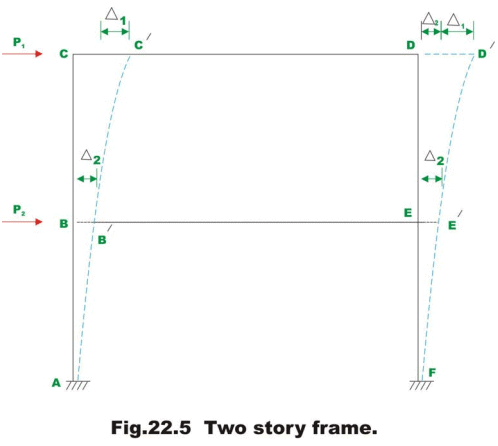Six simultaneous equations are required to evaluate the six unknowns (four rotations and two translations). For each of the member one could write two slope-deflection equations relating beam end moments to (i) externally applied loads and (ii) displacements (rotations and translations). Four of the required six equations are obtained by considering the moment equilibrium of joint B, C, D and E respectively. For example,

∑ MB = 0 ⇒ MBA + MBC + MBE = 0                           (22.1)

The other two equations are obtained by considering the force equilibrium of the members. Thus, the shear at the base of all columns for any story must be equal to applied load. Thus ∑ Fx = 0 at the base of top story gives (ref. Fig. 22.6)

P1 - HC - HD = 0                             (22.2)

Similarly ∑ Fx = 0 at the base of frame results in

P1 + P2 - HA - HF = 0                          (22.3)

Thus we get six equations in six unknowns. Solving the above six equations all the unknowns are evaluated. The above procedure is explained in example 22.1.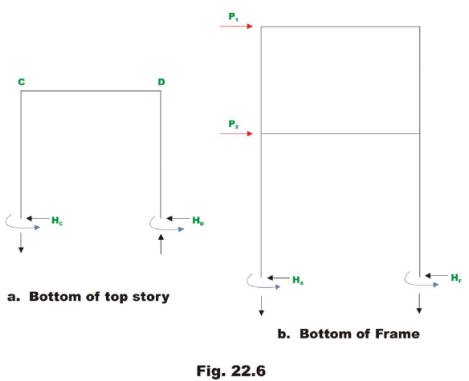Example 22.1

Analyse the two story rigid frame shown in Fig. 22.7a by the slope-deflection method. Assume EI to be constant for all members.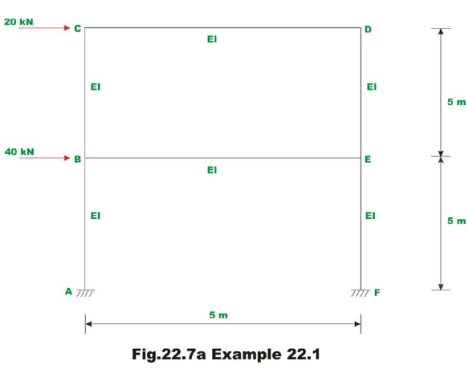In this case all the fixed end moments are zero. The members AB and EF undergo rotations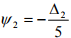(negative as it is clockwise) and member BC and ED undergo rotations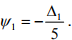Now writing slope-deflection equations for 12 beam end moments.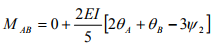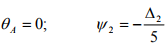MAB = OAEIθB + 0.24EIΔ2

MBA = 08EIθB + 0.24EIΔ2

MBC = 0.8EIθB + 0.4 EIθC + 0.24 EIΔ1

MCB = 0.8EIθC + 0.4EIθB + 0.24EIΔ1

MBE = 0.8EIθB + 0.4EIθE

MEB = 0.8EIθE + 0 AEIθB

MCD = 0.8EIθC + 0.4 EIθD

MDC = 0.8EIθD + 0.4 EIθC

MDE = 0.8EIθD + 0.4EIθE + 0.24EIΔ1

MED = 0.8EIθE + 0.4EIθD + 0.24EIΔ1

MEF = 0.8EIθE + 0.24EIΔ2

MFE = 0.4EIθE + 0.24EIΔ2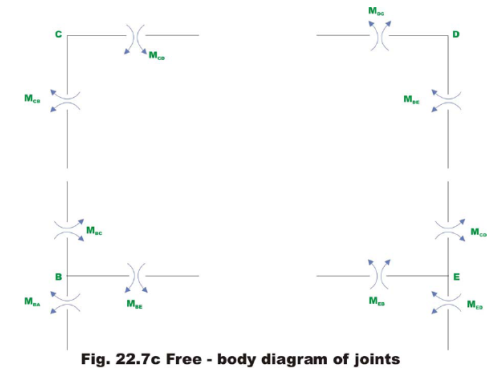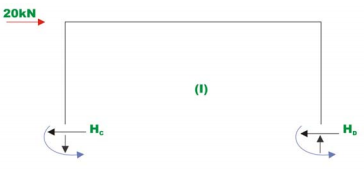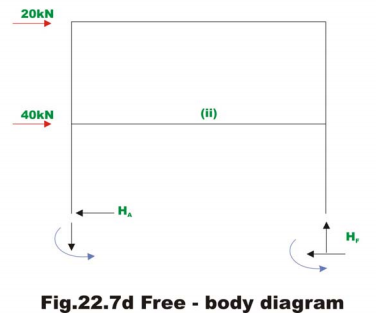Moment equilibrium of joint B, C, D and E requires that (vide Fig. 22.7c).

MBA + MBC + MBE = 0

MCB + MCD = 0

MDC + MDE = 0

MEB + MED + MEF = 0                          (2)

The required two more equations are written considering the horizontal equilibrium at each story level. i.e. ∑ FX = 0 (vide., Fig. 22.7d). Thus,

HC + HD = 20

HA + HF = 60                                (3)

Considering the equilibrium of column AB, EF , BC and ED , we get (vide 22.7c)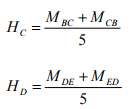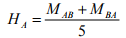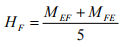(4)

Using equation (4), equation (3) may be written as,

MBC + MCB + MDE + MED = 100

MAB + MBA + MEF + MFE = 300                            (5)

Substituting the beam end moments from equation (1) in (2) and (5) the required equations are obtained. Thus,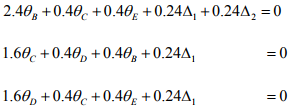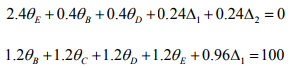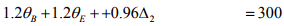(6)

Solving above equations, yields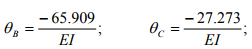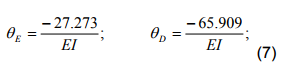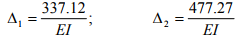Substituting the above values of rotations and translations in equation (1) beam end moments are evaluated. They are,

MAB = 88.18 kN.m ; MBA = 61.81 kN.m

MBC = 17.27 kN.m ; MCB = 32.72. kN.m

MBE = -79.09 kN.m ; MEB = -79.09 kN.m

MCD = -32.72 kN.m ; MDC = -32.72 kN.m

MDE = 32.72 kN.m ; MED = 17.27 kN.m

MEF = 61.81 kN.m; MFE = 88.18 kN.m

Offer running on EduRev: Apply code STAYHOME200 to get INR 200 off on our premium plan EduRev Infinity!

## Structural Analysis

30 videos|122 docs|28 tests

,

,

,

,

,

,

,

,

,

,

,

,

,

,

,

,

,

,

,

,

,

;